• 7.5 MATLAB滤波器设计工具FDATool FDATool(Filter Design and Analysis Tool是MATLAB 信号处理工具箱提供的一种综合简便的图形用户工具通过该工具提供的先进可视化滤波器集成设计环境用户可以方便地设计几乎所有的...
• MATLAB滤波器设计fdatool（filterDesigner）工具箱使用 MATLAB集成了一套功能非常强大的滤波器设计和分析工具FDAtool（新版为filterDesigner），它可以很方便的设计出一个滤波器，并且可以进行性能的分析。 以下操作...
MATLAB滤波器设计fdatool（filterDesigner）工具箱使用
MATLAB集成了一套功能非常强大的滤波器设计和分析工具FDAtool（新版为filterDesigner），它可以很方便的设计出一个滤波器，并且可以进行性能的分析。
以下操作使用的MATLAB环境是MATLAB R2018a
1、启动FDAtool工具箱，在命令行窗口中输入fdatool命令，打开FDAtool工具箱。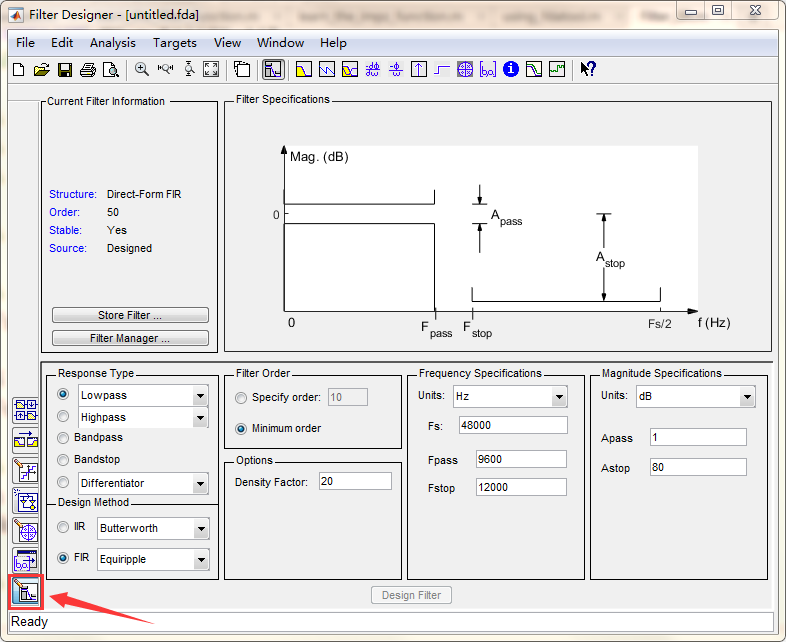注：如果开始界面不是上图所示，则点击红色方框即可。
2、设计界面简单介绍：
1）Response Type（响应类型）：有低通、高通、带通、带阻等设计。
2）Design Method（设计方法）：有IIR和FIR两大类。
3）Filter Order（滤波器阶次）：阶次可以根据自己的需要设定，也可以直接选择最小阶次。
4）Frequency Specifications（频率参数）：根据自己所需要的参数进行设定。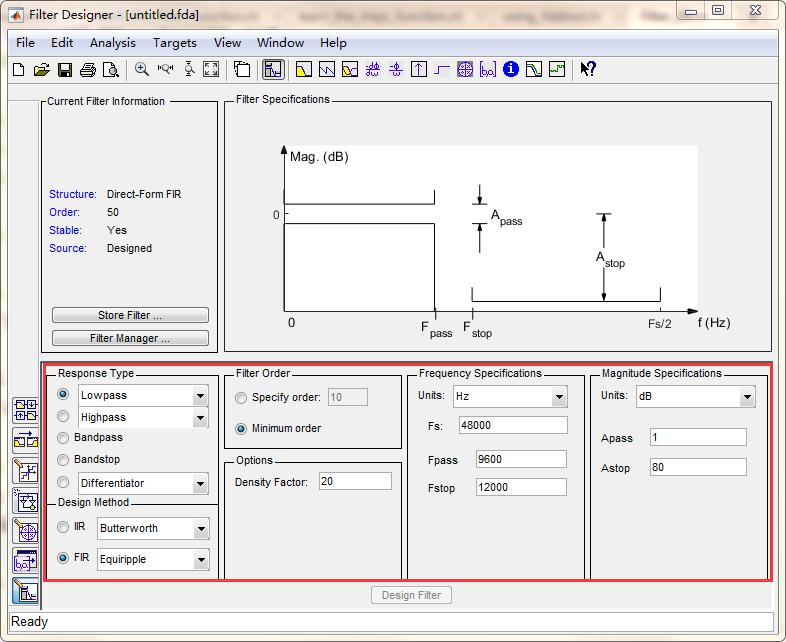例：设计一个一下参数的滤波器：
1）巴特沃斯低通滤波器。
2）阶数为10阶。
3）采样频率为：500Hz。
4）截止频率为：50Hz。
3、根据以上要求设计改变FDAtool工具箱中的参数，并且点击Design Filter，就会显示出所设计的滤波器。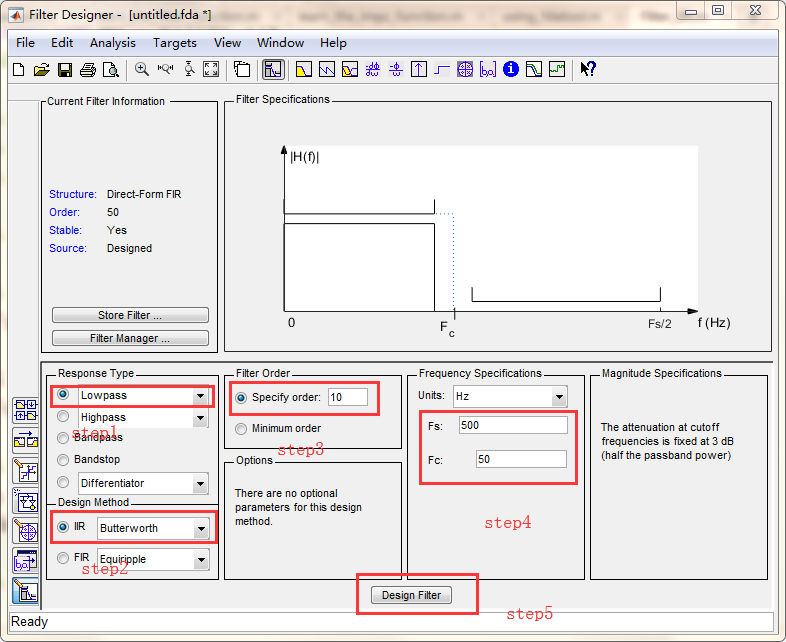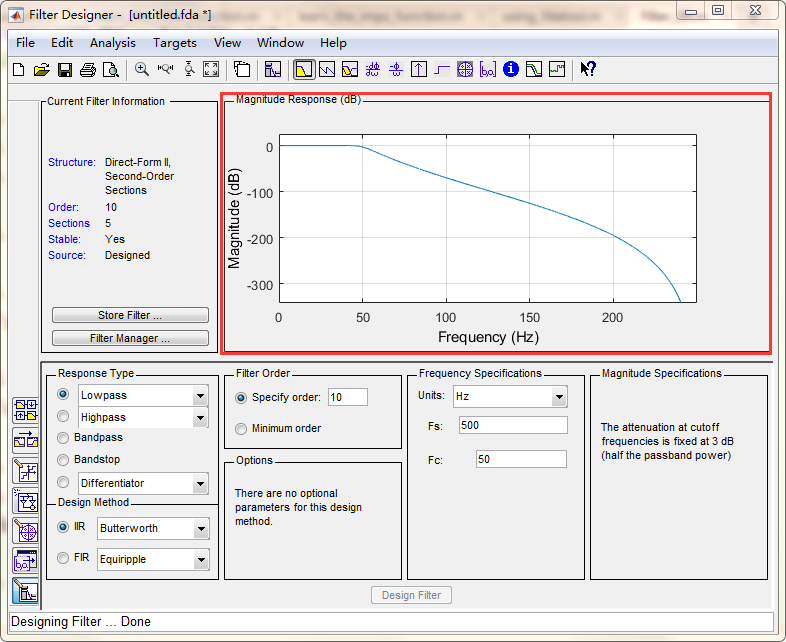4、可以查看滤波器的各项性能：
1）幅度响应：2）相位响应：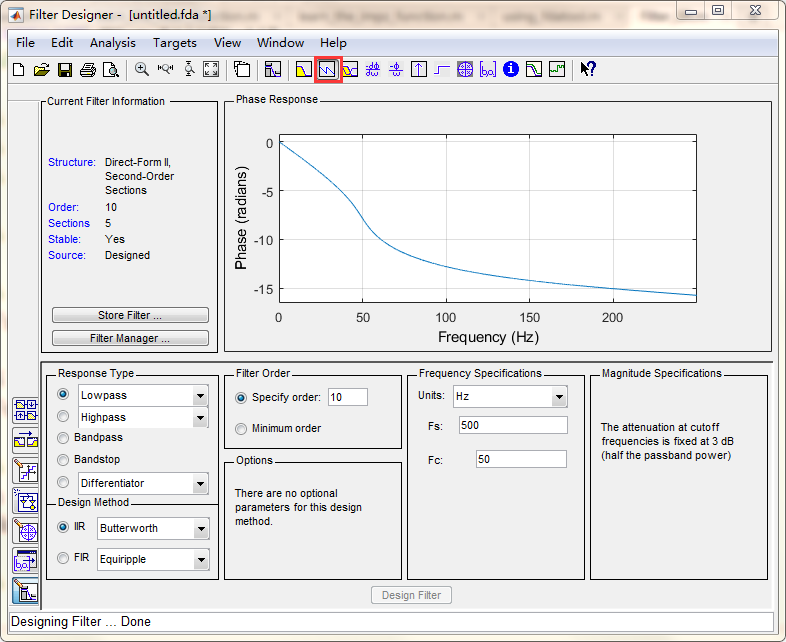3）冲击响应：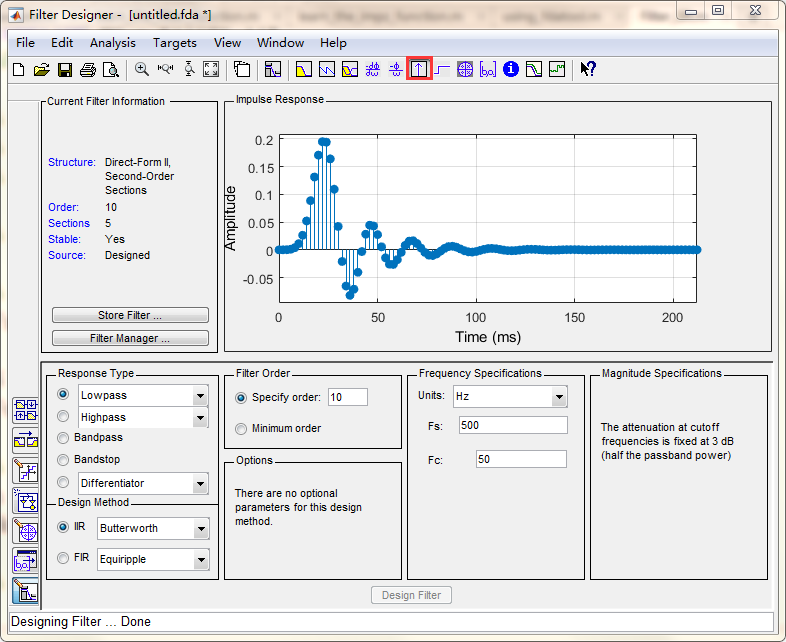4）零极点图：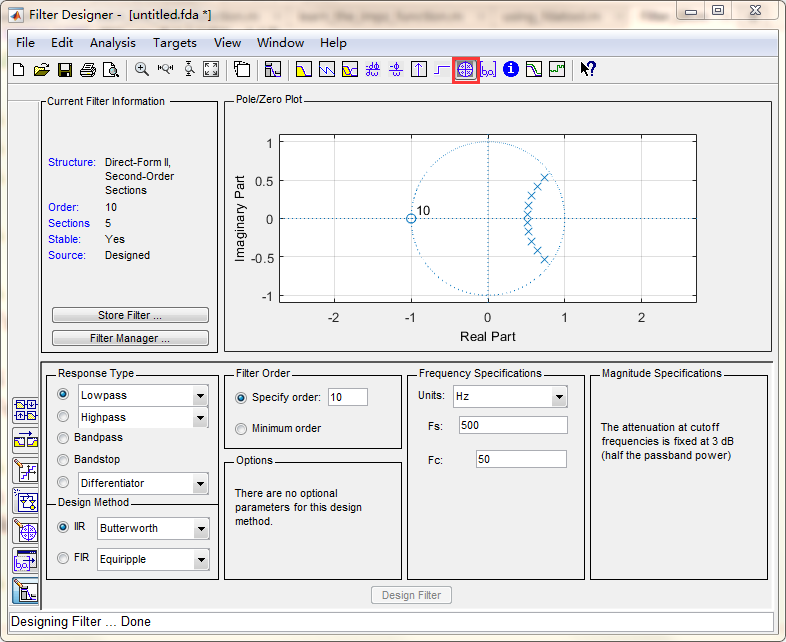5、将设计好的滤波器导出matlab中。
（1）以.m文件的形式导出到matlab中：
1）点击file，然后选择Generate MATLAB Code -> Filter DesignFunction：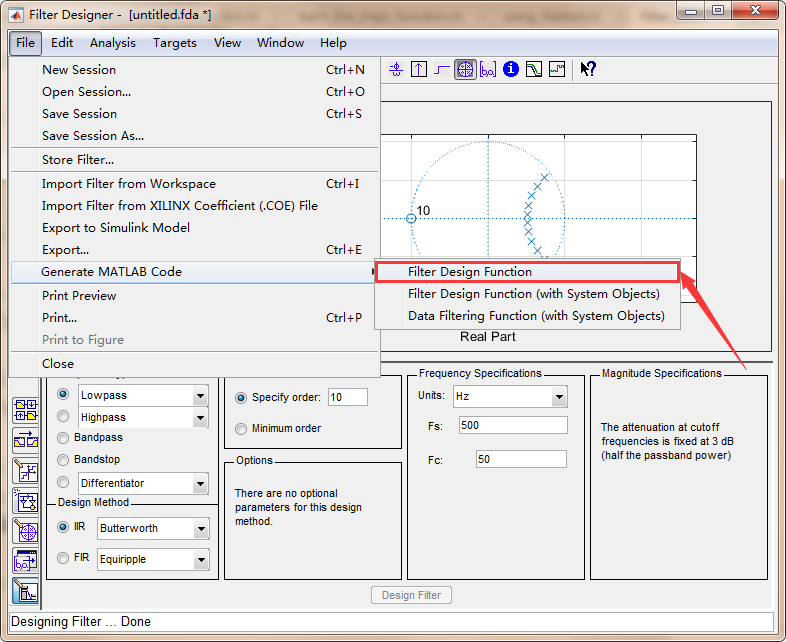2）在当前文件目录中修改导出文件名为Filter_IIR.m，点击保存：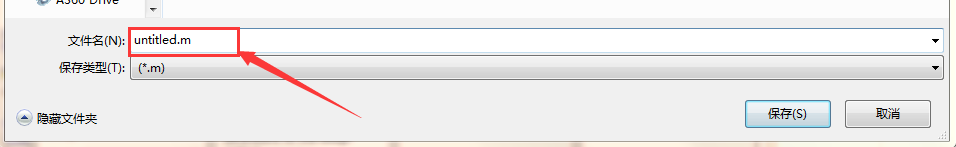3）然后就可以看到已经设计好的滤波器代码。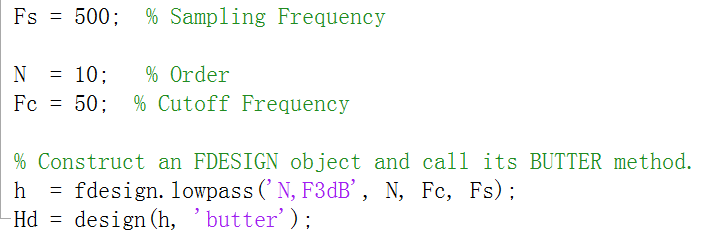4）调用设计好的滤波器：
在MATLAB中新建一个.m文件，然后在文件中添加如下代码：
%%
% 作者：明志
% 环境：MATLAB R2018a
y1 = rand(100,1);
figure('color',[1,1,1]);
subplot(2,1,1);
plot(y1);
title('滤波前波形');
%%
y2 = filter(Filter_IIR,y1);  % 直接使用设计好的滤波器进行滤波，filter函数是滤波函数
subplot(2,1,2);
plot(y2);
title('滤波后波形');

就会得到如下图形：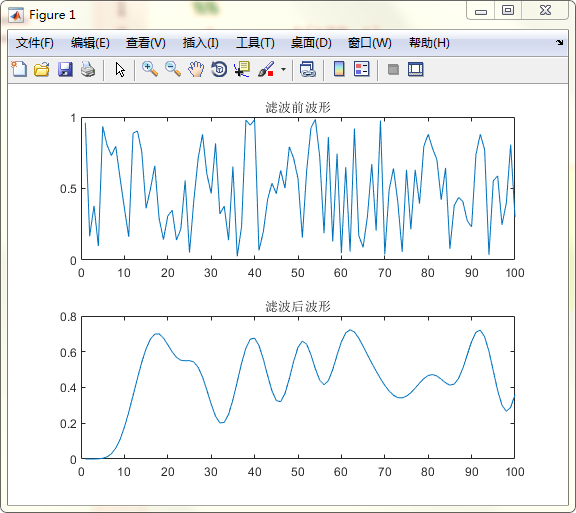（2）将滤波器导出到Workspace中：
1）点击File->Export，然后弹出选择窗口。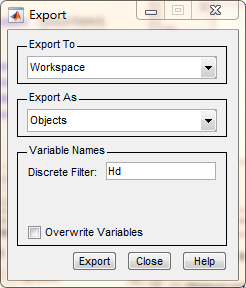2）将Export to选择为Workspace、Export As选择为Object、Variable Names设置为Hd，然后点击Export即可。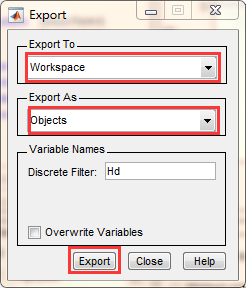3）点击Export之后会在工作区中出现名称为Hd的df2sos变量。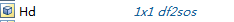4）在命令行窗口中直接输入以下代码即可使用滤波器。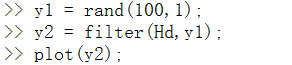滤波前信号为：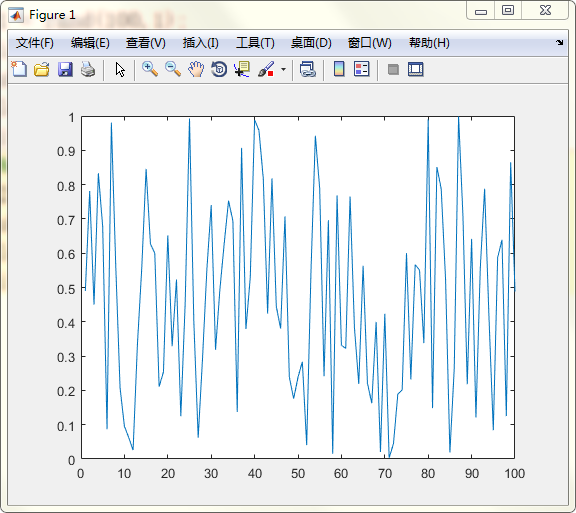滤波后信号为：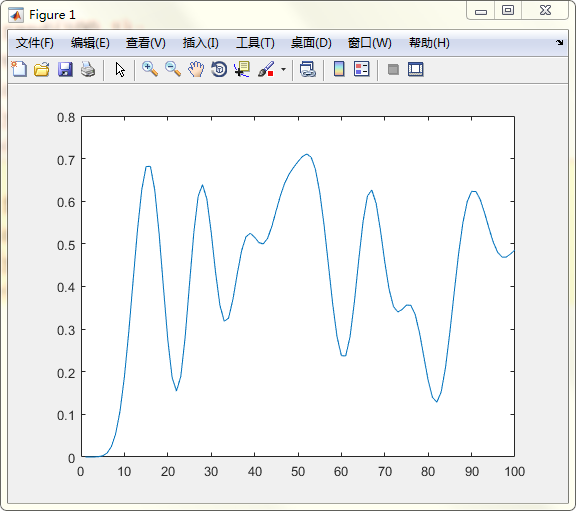对于其他导出方式方法大同小异，只是加载的方式不同而已，读者可以自行尝试。


展开全文• 基本学习使用MATLAB软件中FDAtool工具箱对滤波器设计与分析
• MATLAB滤波器工具，相关设计的一些资料，方便学习下
• 1.搜索工具 2.选择相应的滤波器，填写参数，波纹设计法(Equiripple) 3.Design Filter 生成滤波器系数，File-export 导出系数。
1.搜索工具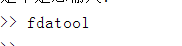2.选择相应的滤波器，填写参数，波纹设计法(Equiripple)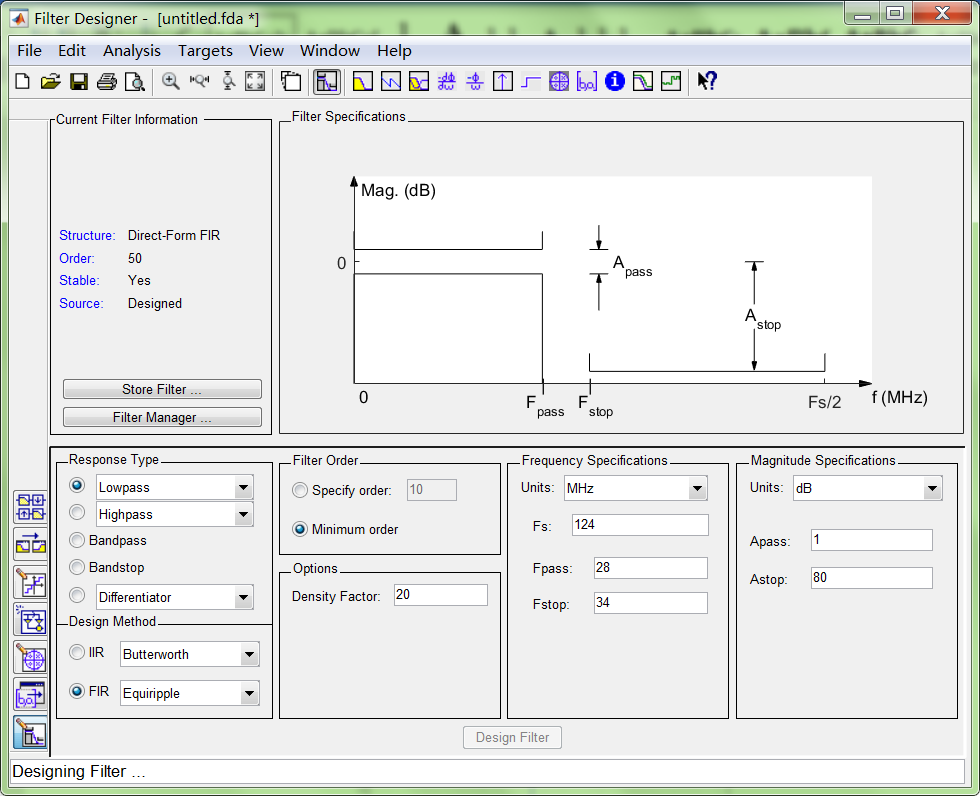3.Design Filter 生成滤波器系数，File-export 导出系数。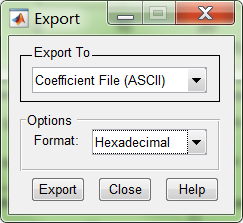展开全文• MATLAB有专门的滤波器设计与分析的工具箱fdatool（Filter Design and Analysis Tool），里面可以很方便地设计各种各样的滤波器，这里介绍下如何简单设计滤波器并进行使用。 在命令栏中输入fdatool PS：fdatool在后续...
MATLAB有专门的滤波器设计与分析的工具箱fdatool（Filter Design and Analysis Tool），里面可以很方便地设计各种各样的滤波器，这里介绍下如何简单设计滤波器并进行使用。
在命令栏中输入fdatool
PS：fdatool在后续的版本中会换成filterDesigner
可以打开fdatool的界面如下所示在界面的左上角是关于设计滤波器的一些信息，包括设计滤波器的结构、阶数，稳定性和设计状态。右上角是关于滤波器的幅频响应（默认），通过Analysis可以得到其他曲线，如相频响应、群延时、零极点等等。界面的下方是关于滤波器的具体参数，包括响应类型、设计方法、采样率、滤波器阶数、截止频率、通带，阻带波动等等可以看出有低通、高通、带通、带阻类型的滤波器，以及是FIR还是IIR滤波器，具体类型及数值可以根据设计的要求进行选定，这里就不再叙述。
下面给出一个简单的例子。
对于信号
$y={{s}_{1}}\text{+}{{s}_{2}}+{{s}_{3}}$
其中，${{s}_{1}}=\sin \left( 2\pi {{f}_{1}}t \right)$，${{s}_{2}}=\sin \left( 2\pi {{f}_{2}}t \right)$，${{s}_{3}}=\sin \left( 2\pi {{f}_{3}}t \right)$，${{f}_{1}}=60\text{Hz}$ ，${{f}_{2}}=130\text{Hz}$，${{f}_{3}}=1000\text{Hz}$，要求设计一个低通滤波器，滤除频率130Hz和1000Hz的信号。
我们可以按照要求很轻易地设计出一个滤波器为了能够调用这个滤波器，我们可以将其保存成一个函数如myfilter.m，这样后续就可以直接调用，但是这种调用方式相当于在运行到该命令时重新设计滤波器，对于一些阶数比较大的滤波器可能会耗时比较长。保存的函数为
function Hd = myfilter
%MYFILTER Returns a discrete-time filter object.

% MATLAB Code
% Generated by MATLAB(R) 9.1 and the Signal Processing Toolbox 7.3.
% Generated on: 07-Feb-2021 11:37:07

% Butterworth Lowpass filter designed using FDESIGN.LOWPASS.

% All frequency values are in Hz.
Fs = 10000;  % Sampling Frequency

Fpass = 80;          % Passband Frequency
Fstop = 90;          % Stopband Frequency
Apass = 0.1;         % Passband Ripple (dB)
Astop = 80;          % Stopband Attenuation (dB)
match = 'stopband';  % Band to match exactly

% Construct an FDESIGN object and call its BUTTER method.
h  = fdesign.lowpass(Fpass, Fstop, Apass, Astop, Fs);
Hd = design(h, 'butter', 'MatchExactly', match);

% [EOF]

也可以直接导出滤波器的系数，这样就可以直接调用系数进行滤波，会加快程序运行的速度。具体在：File——Export，界面如下：可以看出，系数可以导出ASCII码（可以用于硬件如DSP、FPGA等）、.mat文件等等，这里就不再叙述。
利用MATLAB画出原始信号为利用该滤波器对上述信号进行滤波可以得到如下从图中可以看出，经过滤波器滤除之后的信号基本能够很好地恢复出原始的信号，从信号的频谱也能够看出，130Hz和1000Hz的信号被滤除。图中的频谱结果有一些幅度有一些衰减，这是正常的，同时频谱没有原始信号的频谱光滑，这是滤波器的阶数造成的，可以通过增加滤波器的阶数进行改善。
PS：由于滤波器存在一定的建立时间，即非理想滤波器，滤波之后的信号在刚开始的时间和后面的是不一致的，这里显示的是0.2s之后的信号。
代码如下：
clear;
close all;
clc;
f1=60;f2=130;f3=1e3;          %原始信号频率
fs=1e4;                       %采样频率
Ns=1e4;                       %采样点数
t=0:1/fs:Ns/fs;
s1=sin(2*pi*f1*t);
s2=sin(2*pi*f2*t);
s3=sin(2*pi*f3*t);
figure(1);
subplot(211)
plot(t,s1,'r',t,s2,'k',t,s3,'g');
xlim([0 500/fs])
title('正弦信号');xlabel('t/s');
y=s1+s2+s3;
set(gcf,'color','w');
subplot(212)
plot(t,y);
title('原始信号');xlabel('t/s');
set(gcf,'color','w');
N=4096;
f=(0:N-1)*fs/N;
yy=abs(fft(y,N)*2/N);
figure(2);
subplot(311)
plot(f(1:N/2),yy(1:N/2));
title('原始信号的FFT');xlabel('f/Hz');
xlim([0 1500]);
h=myfilter;
ylp=filter(h,y);
subplot(312)
plot(t,ylp);
xlim([0.2 0.8]);
title('低通滤波之后的信号')
xlabel('t/s');
yyy=abs(fft(ylp,N)*2/N);
subplot(313)
plot(f(1:N/2),yyy(1:N/2));
xlabel('f/Hz');
title('低通滤波之后的信号频谱');
xlim([0 1500]);



展开全文• 有频率为5Hz、15Hz、30Hz的叠加余弦信号，设计低通滤波器保留5Hz的频率分量，设计高通滤波器保留30Hz的频率分量，设计带通滤波器保留20Hz的频率分量，设计带阻滤波器滤除5Hz和30Hz的频率分量。 一、pandas是什么？ ...
文章目录问题提出一、低通滤波器1.保留5Hz2.运行结果二、高通滤波器1.保留30Hz2.运行结果三、带通滤波器1.保留20Hz2.运行结果四、带阻滤波器1.滤除5Hz和30Hz2.运行结果最后

问题提出
有频率为5Hz、15Hz、30Hz的叠加余弦信号，进行如下设计：
（1）设计低通滤波器保留5Hz的频率分量；
（2）设计高通滤波器保留30Hz的频率分量；
（3）设计带通滤波器保留20Hz的频率分量；
（4）设计带阻滤波器滤除5Hz和30Hz的频率分量。

一、低通滤波器
1.保留5Hz
clear
clc
f1=5;%第一个点频信号分量频率
f2=15;%第二个点频信号分量频率
f3=30;%第三个点频信号分量频率
fs=150;%采样率
T=2;%时宽
B=10;%FIR截止频率
n=round(T*fs);%采样点个数
t=linspace(0,T,n);
y=cos(2*pi*f1*t)+cos(2*pi*f2*t)+cos(2*pi*f3*t);%叠加信号
figure;
subplot(221)
plot(t,y);
title('原始信号时域');
xlabel('t/s');
ylabel('幅度');
fft_y=fftshift(fft(y));%将fft结果以fs/2为中心左右互换
f=linspace(-fs/2,fs/2,n);
subplot(222)
plot(f,abs(fft_y));
title('原始信号频谱');
xlabel('f/Hz');
ylabel('幅度');
axis([ 0 50 0 100]);
b=fir1(80, B/(fs/2),'low'); %低通
y_after_fir=filter(b,1,y);%
subplot(223)
plot(t,y_after_fir);
title('滤波后信号时域');
xlabel('t/s');
ylabel('幅度');
fft_y1=fftshift(fft(y_after_fir));%将fft结果以fs/2为中心左右互换
f=linspace(-fs/2,fs/2,n);
subplot(224)
plot(f,abs(fft_y1));
title('滤波后信号频谱');
xlabel('f/Hz');
ylabel('幅度');
axis([ 0 50 0 100]);
figure;
freqz(b);%数字滤波器频率响应

2.运行结果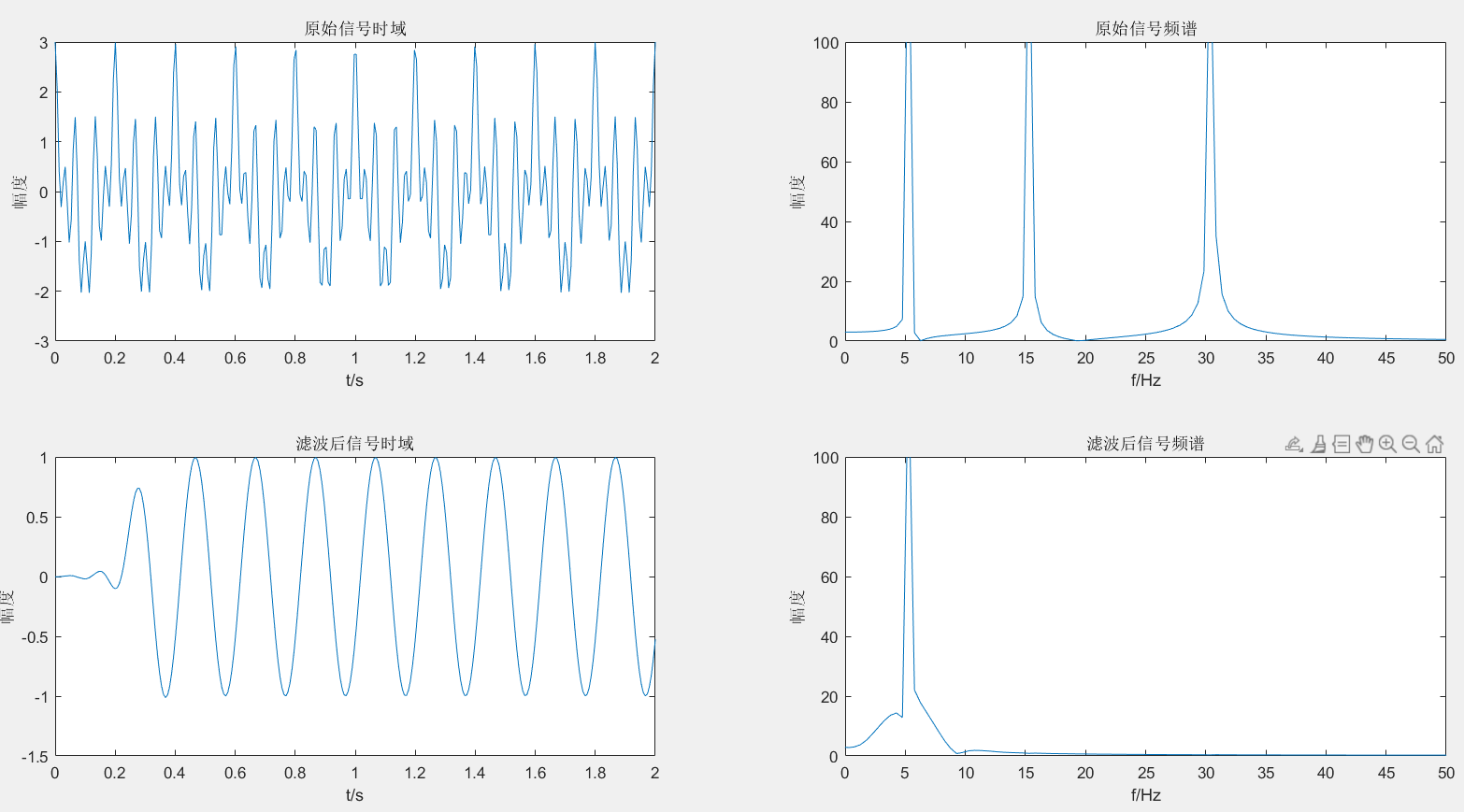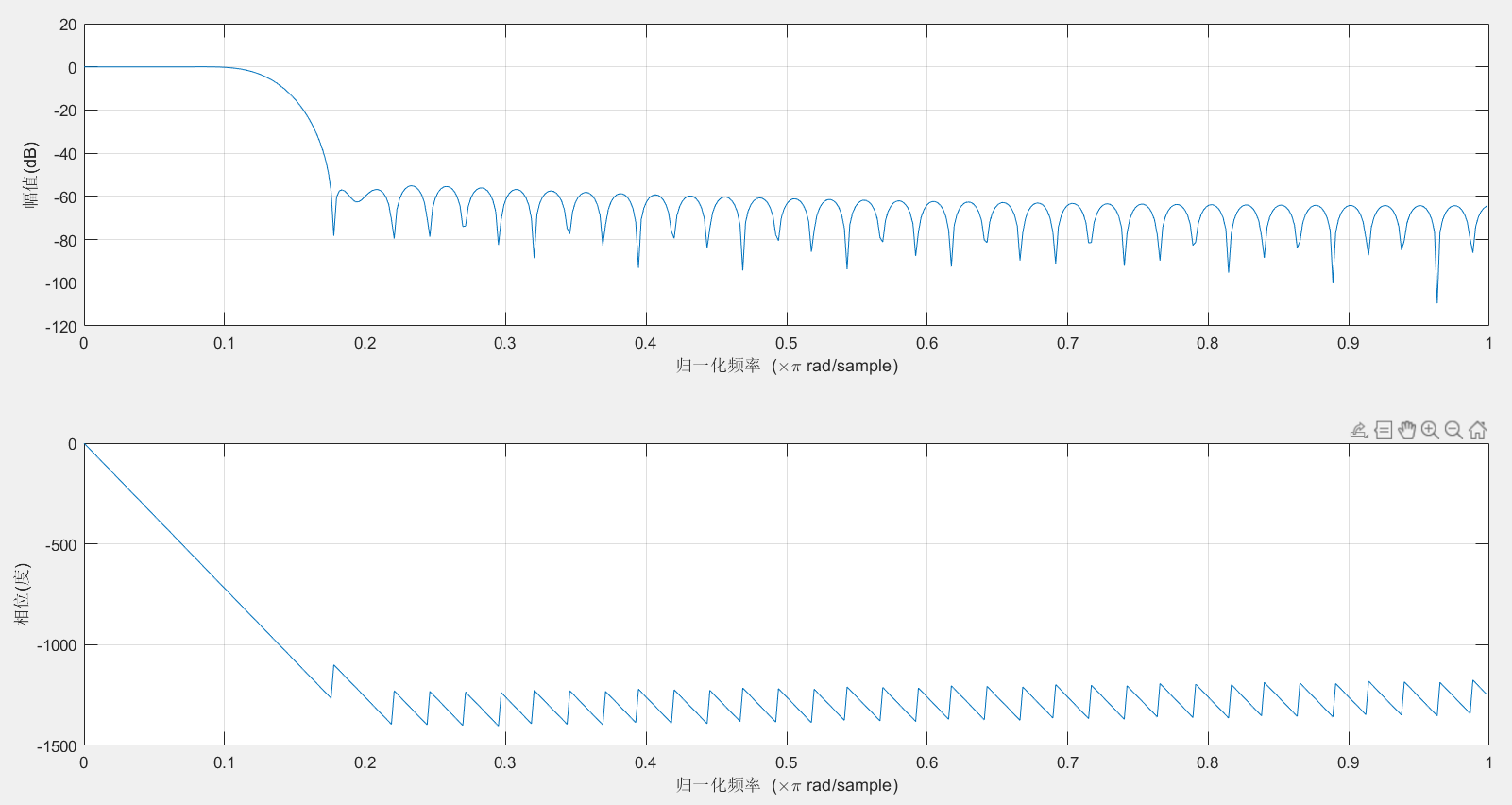二、高通滤波器
1.保留30Hz
clear
clc
f1=5;%第一个点频信号分量频率
f2=15;%第二个点频信号分量频率
f3=30;%第三个点频信号分量频率
fs=150;%采样率
T=2;%时宽
B=25;%FIR截止频率
n=round(T*fs);%采样点个数
t=linspace(0,T,n);
y=cos(2*pi*f1*t)+cos(2*pi*f2*t)+cos(2*pi*f3*t);
figure;
subplot(221)
plot(t,y);
title('原始信号时域');
xlabel('t/s');
ylabel('幅度');
fft_y=fftshift(fft(y));
f=linspace(-fs/2,fs/2,n);
subplot(222)
plot(f,abs(fft_y));
title('原始信号频谱');
xlabel('f/Hz');
ylabel('幅度');
axis([ 0 50 0 100]);
b=fir1(80, B/(fs/2),'high'); %高通
y_after_fir=filter(b,1,y);
subplot(223)
plot(t,y_after_fir);
title('滤波后信号时域');
xlabel('t/s');
ylabel('幅度');
fft_y1=fftshift(fft(y_after_fir));
f=linspace(-fs/2,fs/2,n);
subplot(224)
plot(f,abs(fft_y1));
title('滤波后信号频谱');
xlabel('f/Hz');
ylabel('幅度');
axis([ 0 50 0 100]);
figure;
freqz(b);%数字滤波器频率响应

2.运行结果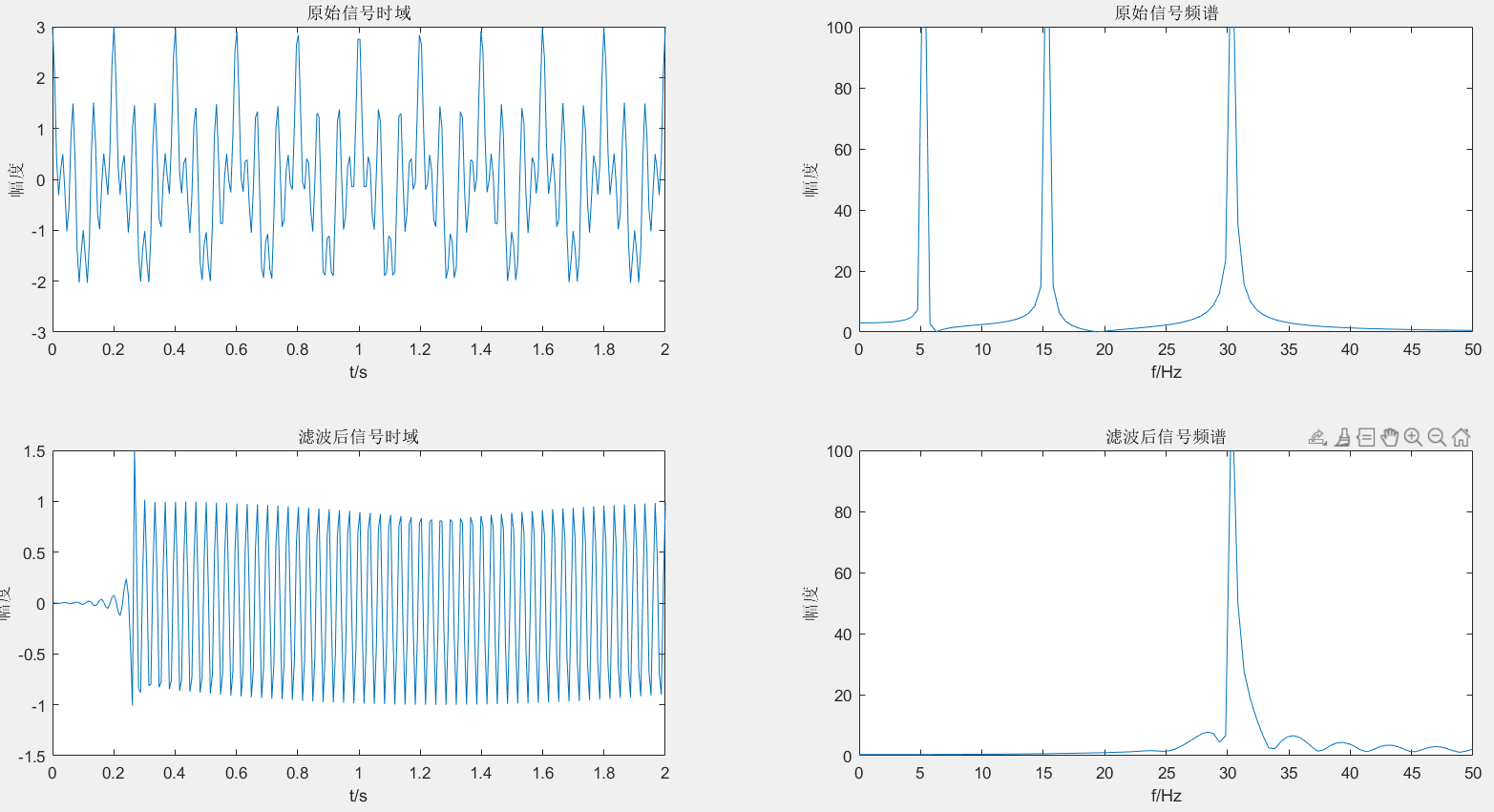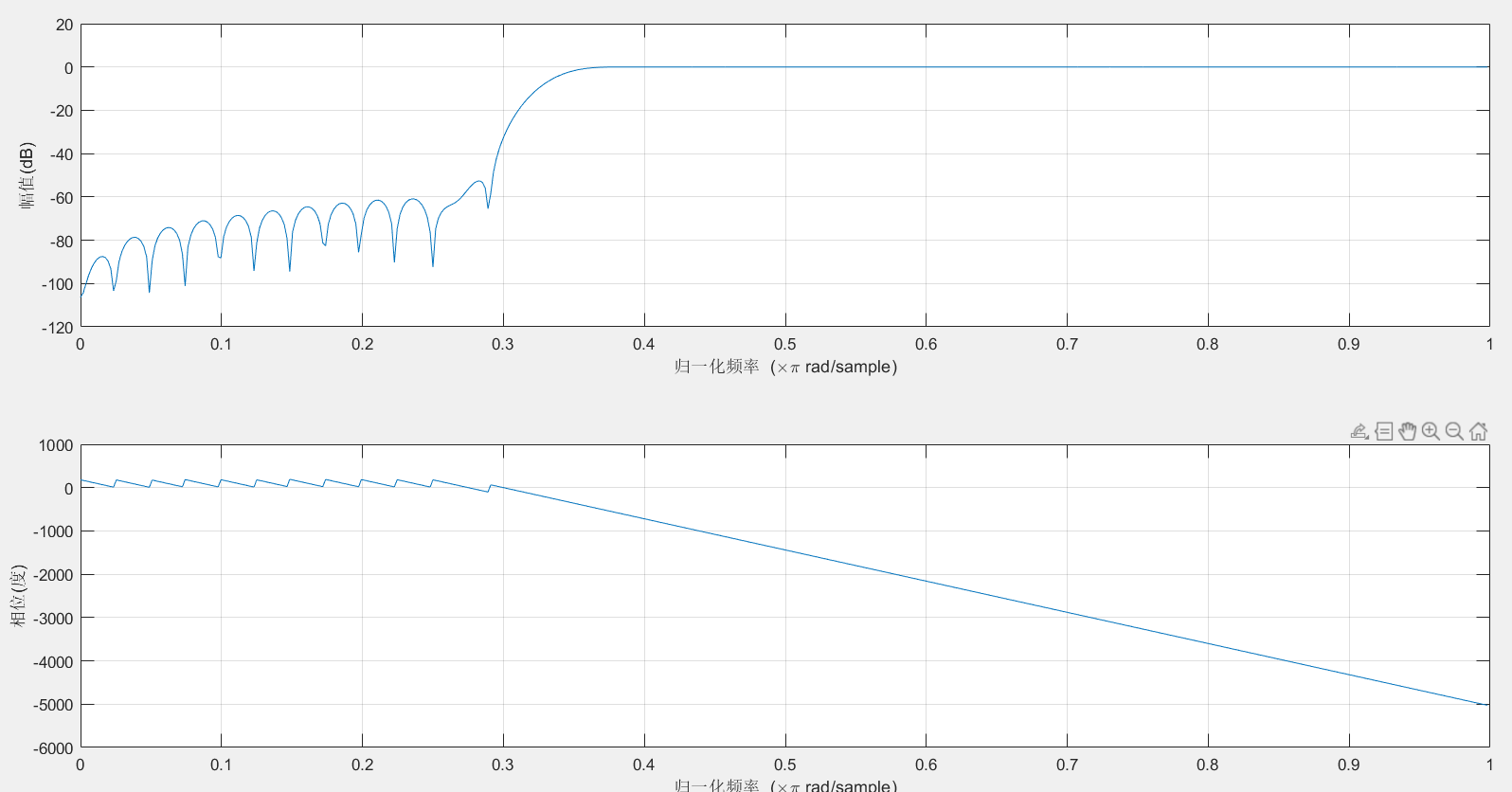三、带通滤波器
1.保留20Hz
clear
clc
f1=5;%第一个点频信号分量频率
f2=15;%第二个点频信号分量频率
f3=30;%第三个点频信号分量频率
fc1=10;
fc2=25;
fs=150;%采样率
[n,Wn,beta,ftype]=kaiserord([7 13 17 23] ,[0 1 0], [0.01 0.01 0.01] ,100) ;
w1=2*fc1/fs; w2=2*fc2/fs ;
window=kaiser(n+1 ,beta) ;%使用kaiser窗函数
b=fir1 (n, [w1 w2],window) ;%使用标准频率响应的加窗设计函数fir1
T=2;%时宽
n=round(T*fs);%采样点个数
t=linspace(0,T,n);
s = cos(2*pi*t*f1) +cos (2*pi*t*f2) +cos (2*pi*t*f3) ;
sf = filter (b,1,s) ;%对信号s进行滤波
f=linspace(-fs/2,fs/2,n);
S=fftshift(fft(s)) ;
SF=fftshift(fft(sf)) ;
figure
subplot(2,2,1) ;
plot(t,s)
%画出时域内的号
subplot(2,2,3) ;
plot(t,sf)
%画出时域内的信号
subplot(2,2,2) ;
f1=abs(S);
f2=abs(SF);
plot(f,f1) ; %画出频域内的信号
title('原始信号频谱');
axis([ 0 50 0 100]);
subplot(2,2,4) ;
plot(f,f2);%画出频域内的信号
title('滤波后信号频谱');
axis([ 0 50 0 100]);
figure;
freqz (b,1,512) ;%数字滤波器频率响应

2.运行结果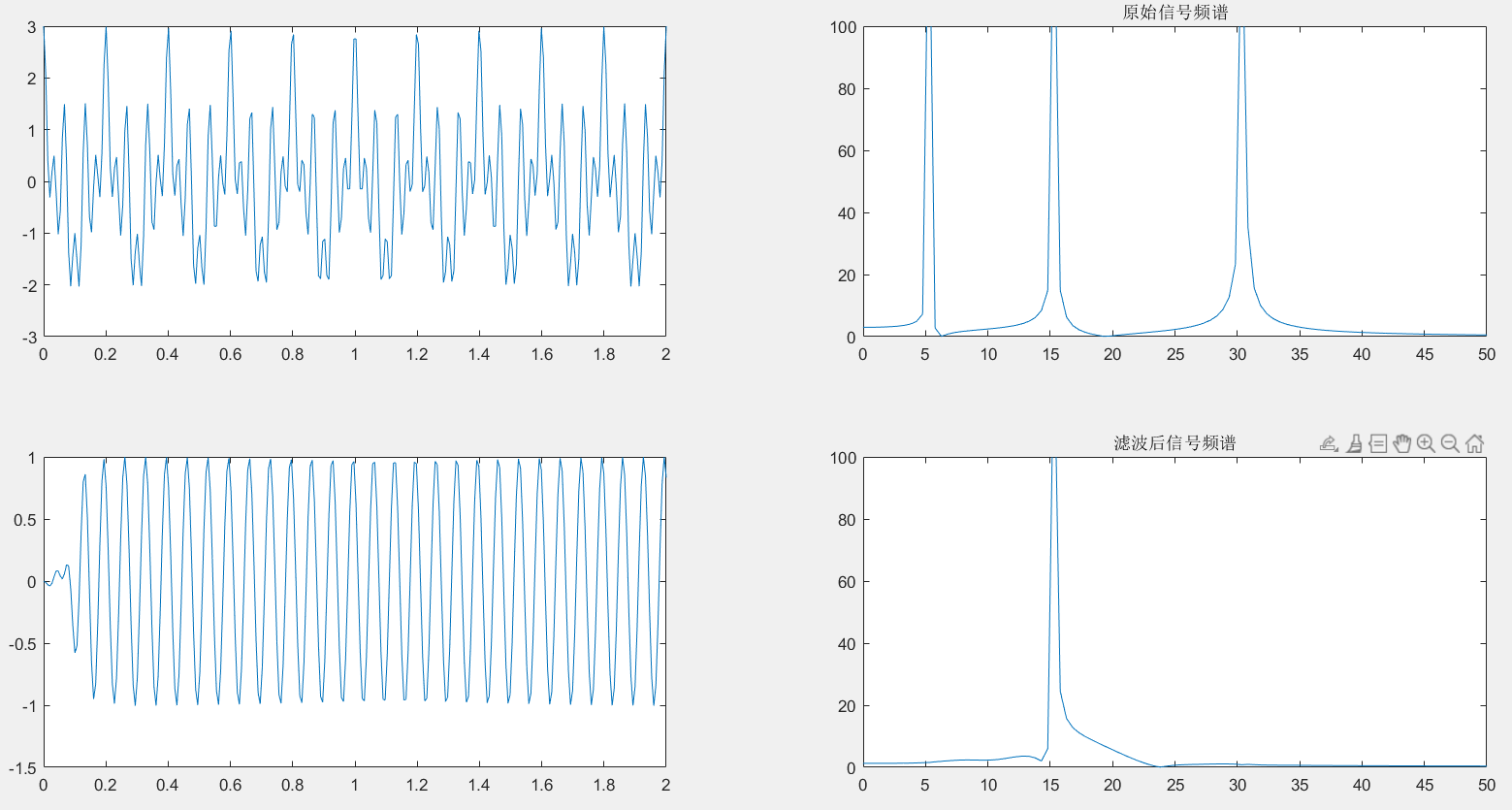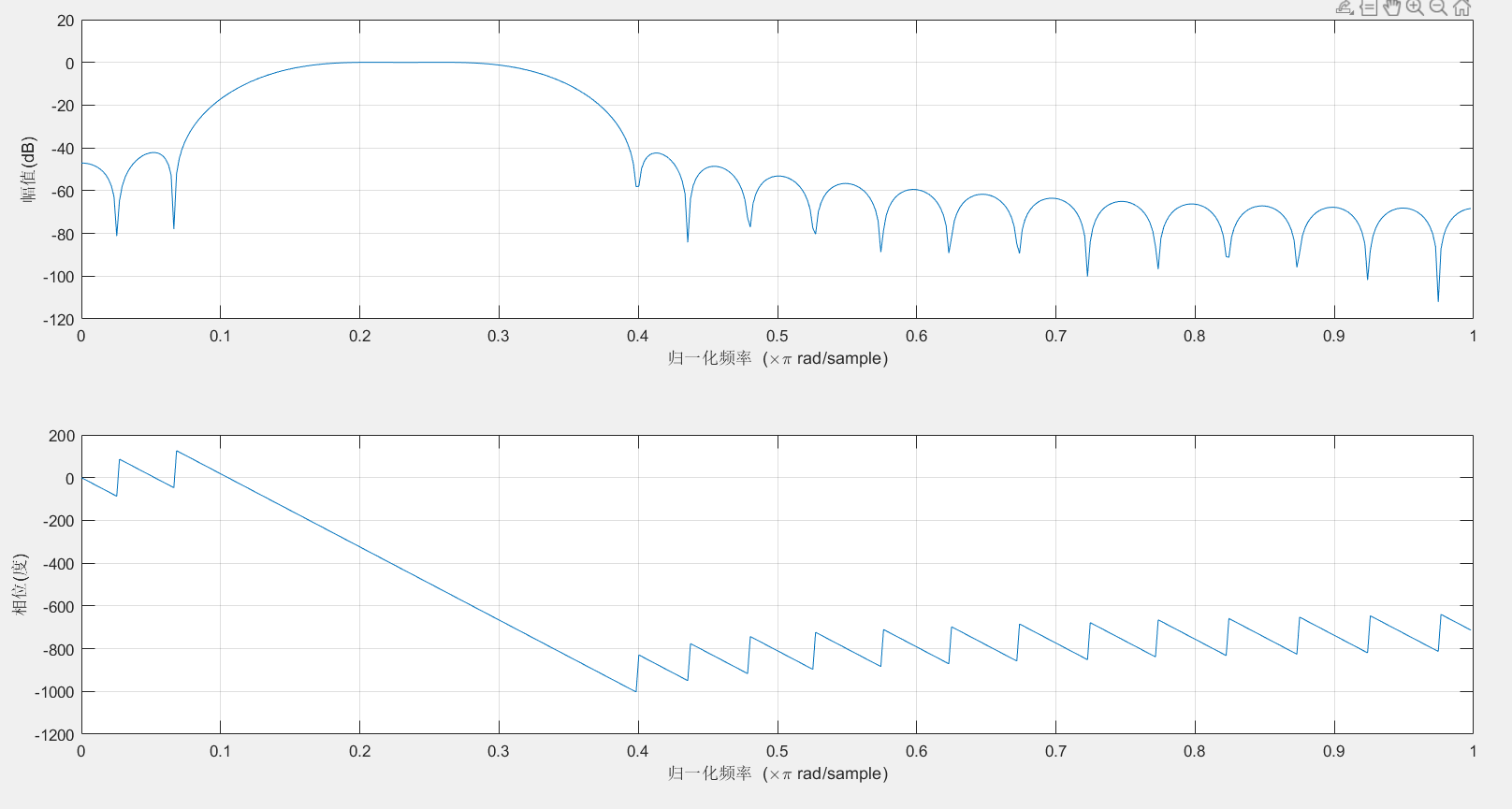四、带阻滤波器
1.滤除5Hz和30Hz
clear
clc
f1=5;%第一个点频信号分量频率
f2=15;%第二个点频信号分量频率
f3=30;%第三个点频信号分量频率
fs=150;
T=2;%时宽
n=round(T*fs);%采样点个数
t=linspace(0,T,n);
y=cos(2*pi*f1*t)+cos(2*pi*f2*t)+cos(2*pi*f3*t);
flp=0;
fhp=30;
fls=15;
fhs=25;
wlp=2*pi*flp/fs;
whp=2*pi* fhp/fs;
wls=2*pi*fls/fs;
whs=2*pi* fhs/fs;
wc=[(wlp+wls)/(2* pi),(whp+whs)/(2*pi)];
delta1=wls-wlp;
delta2=whp-whs;
delta_w=min(delta1 ,delta2);
N=ceil(1.8*pi/delta_w); %不同的窗要选择系数不同
N=N+rem(N,2);
window=boxcar(N+1); %选择窗函数
b=fir1 (28, wc,window) ;
ylb=filter(b,1,y);
f=linspace(-fs/2,fs/2,n);
y1=fftshift(fft(y));
ylb1=fftshift(fft(ylb)) ;
figure(1)
subplot(221)
plot(t,y)
subplot(222)
y1=abs(y1);
plot(f,y1)
title('原始信号频谱');
axis([ 0 50 0 100]);
subplot(223)
plot(t,ylb)
subplot(224)
ylb1=abs(ylb1);
plot(f,ylb1)
title('滤波后信号频谱');
axis([ 0 50 0 100]);
figure
freqz (b,1,512) ;%数字滤波器频率响应


2.运行结果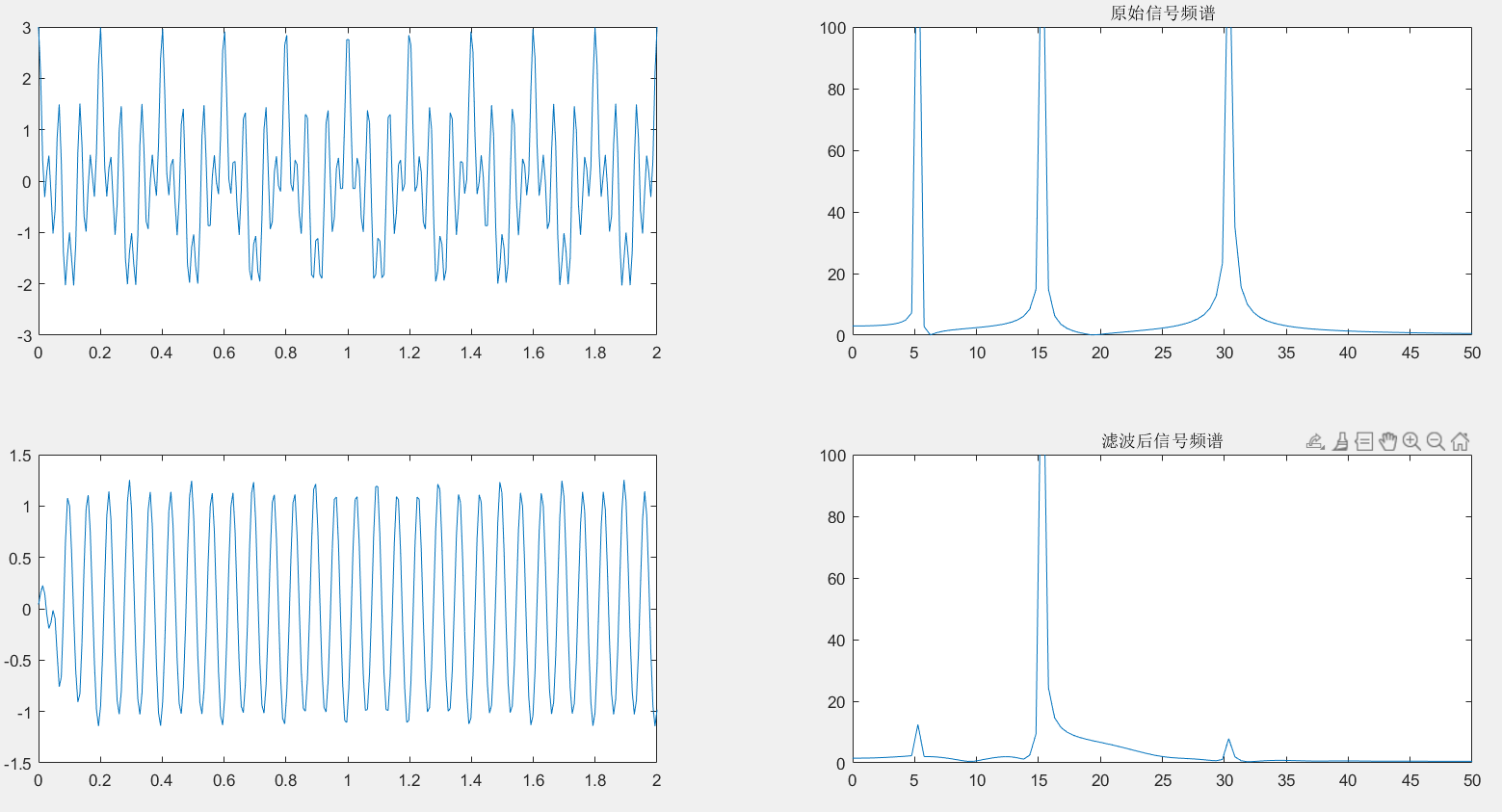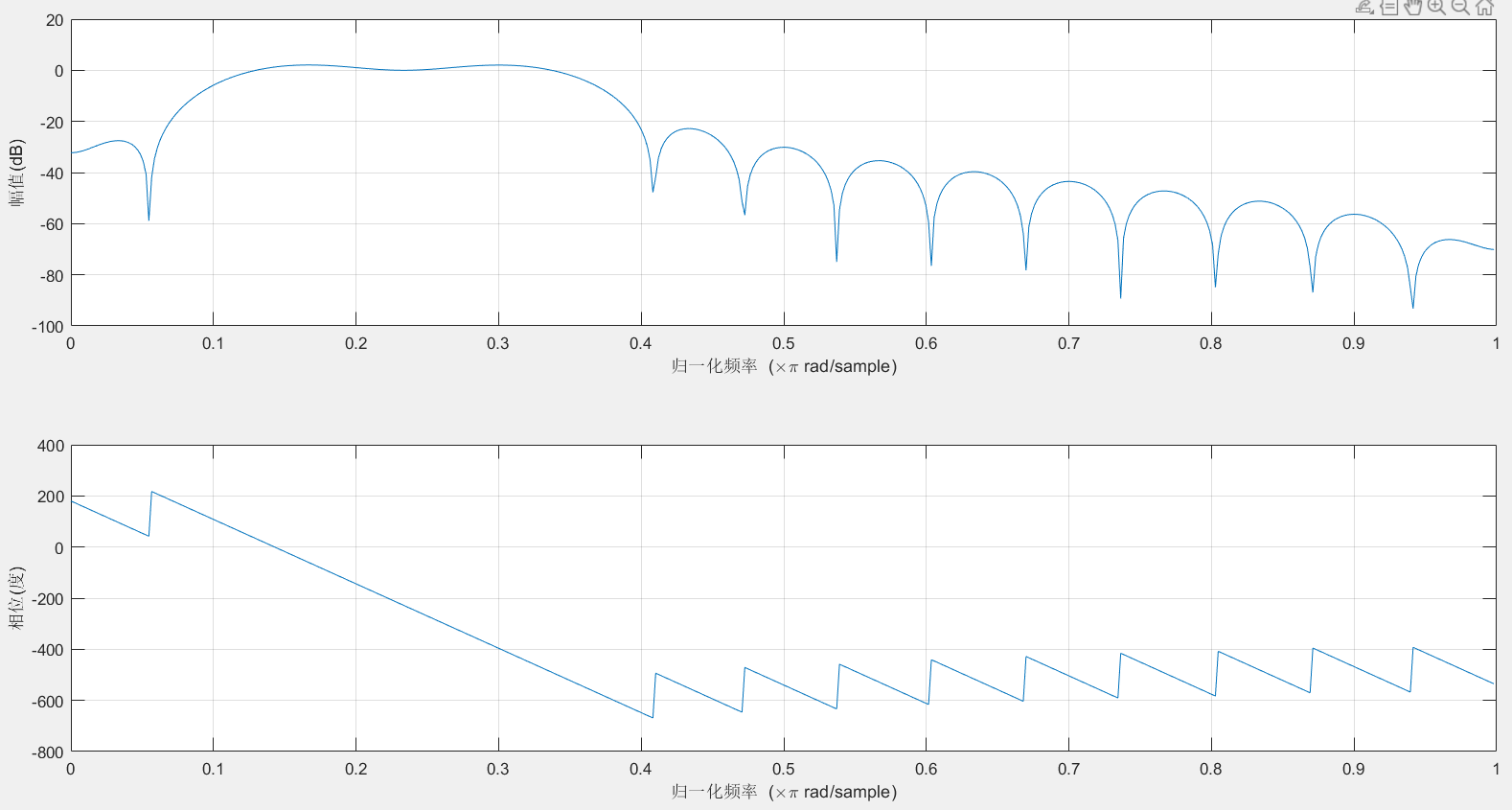最后
不定期发布一些matlab设计内容,敬请期待。包括但不限于如下内容:信号处理、通信仿真、gui设计、matlab appdesigner，simulink仿真。有任何有关MATLAB的问题可加QQ：2802009708进行咨询。或扫码进行添加。展开全文数字信号处理 filter
• 详细介绍matlab滤波器设计工具箱的使用，并通过实例设计一给定指标的数字滤波器
• 基于MATLAB信号处理工具箱的数字滤波器设计与仿真-E5处理工具箱的数字滤波器设计与仿真.zip 摘要：传统的数字滤波器的设计过程复杂，计算工作量大，滤波特性调整困难，影响了它的应用。本文介绍了一种利用MATLAB...
• 以99阶FIR低通滤波器为例，学习使用matlab的fdatool工具箱设计滤波器，并将滤波器系数导出到.coe文件，联合Vivado进行FPGA的FIR滤波器设计。 本文滤波器参数为：低通FIR滤波器，窗函数设计，采用布莱克曼窗，99阶，...
• 然后，为了验证fir滤波器的级联情况，又设计了一个1000阶的fir滤波器对上面的滤波结果进行再次滤波，滤波器设计界面如下： 图：第二级fir滤波器设计界面： 可以得到下面的时域波形。 图表5：经过4000阶低通fir之后...
• MATLAB信号处理工具箱进行 FIR 滤波器设计的三种方法 摘 要 介绍了利用 MATLAB 信号处理工具箱进 行 FIR 滤波器设计的三种方法程序设计法 FDATool 设计法和 SPTool 设计法给出了详 细的设计步骤 并将设计的滤波器...
• 基于matlab的fdatool工具箱的数字滤波器设计详解总结_zhb 基于matlab的fdatool工具箱的数字滤波器设计详解总结_zhb.pdf 基于matlab的fdatool工具箱的数字滤波器设计详解总结_zhb
• 摘 要 介绍了利用 MATLAB 信号处理工具箱进行 FIR 滤波器设计的三种方法程序设计法 FDATool 设计法和 SPTool 设计法给出了详细的设计步骤并将设计的滤波器应用到一个 混和正弦波信号以验证滤波器的性能 关键词 ...
• Matlab Fdatool滤波器设计以及使用 1.命令行中键入fdatool。或者在App导航栏中找到Filter Designer。 2.打开滤波器工具后，选择滤波器的功能，FIR,IIR，等。设置后滤波器参数后，点击下方的Design Filter按钮...
• 基于 MATLAB 信号处理工具箱的数字滤波器设计与仿真.txt 逆风的方向更适合飞翔我不 怕万人阻挡只怕自己投降你发怒一分钟便失去 60 分钟的幸福忙碌是一种幸福让我 们没时间体会痛苦;奔波是一种快乐让我们真实地感受...
• 以99阶FIR低通滤波器为例，学习使用matlab的fdatool工具箱设计滤波器，并将滤波器系数导出到.coe文件，联合Vivado进行FPGA的FIR滤波器设计。 本文滤波器参数为：低通FIR滤波器，窗函数设计，采用布莱克曼窗，99阶，...fpga 数字通信 数字信号处理
• 基于 matlab 的低通滤波器 摘要:调用 MATLAB 信号处理...标 设计过渡模拟滤波器将过渡模拟滤波器系统函数转换成数字滤波器的系统函数MATLAB 信号处理工 具箱中的各种 IIR 数字滤波器设计函数都是采用双线性变换法 关
• 今天给大侠带来基于Matlab中FDATool工具箱的滤波器设计及相关文件的生成，话不多说，上货。 一、摘要 除了采用编程的方法实现滤波器之外，Matlab中自带工具箱FDATool也能很方便快速的实现滤波器的设计。另外...fpga
• MATLAB 中用FDATool 设计滤波器及使用 该文章讲述了 MATLAB 中用FDATool 设计滤波器及使用. 1. 在 Matlab 中键入 fdatool 运行 Filter Design and Analysis Tool具 体使用请参见 Matlab Help 中的Signal Processing ...
• 前言今天要对一之前...分析方法1先将系数导入MATLAB自带的fdatool工具中，工具会自动绘制出滤波器的幅频和相频特性。步骤为：将系数B存入到MATLAB工作区中。打开fdatool，“file”-"inport filter from workspace...matlab 滤波器
• 利用MATLAB设计滤波器，可以随时对比设计要求和滤波器特性调整参数，直观简便，极大地减轻了工作量，有利于滤波器设计的最优化。还介绍了如何利用MATLAB环境下的仿真软件Simulink对所设计的滤波器进行模拟仿真。
• 基于MATLAB工具箱的单片机信号采集滤波器设计，许景波，吴云，在以单片机为核心的信号采集与处理系统中，往往存在着各种干扰信号，严重影响了测量结果和数据的评定。滤波器一直是抑制噪声的有
• 要 介绍了利用TLAB信号处理工具箱进行FI滤波器设计的三种方法程序设计法FDTo设计法和Pol设计法,给出了详细的设计步骤并将设计的滤波器应用到一个混和正弦波信号以验证滤波器的性能 关键词? MTLAB,数字滤波器,有限...
• 文中针对传统FIR滤波器设计方法繁琐，设计步骤和过程繁杂，且设计好滤波器之后，不能直观快速修改滤波器参数来观察滤波器变化，设计了基于MATLAB的FIR数字滤波器。MATLAB功能强大，在进行有限脉冲响应滤波器（FIR）......

# matlab滤波器设计工具matlab 订阅